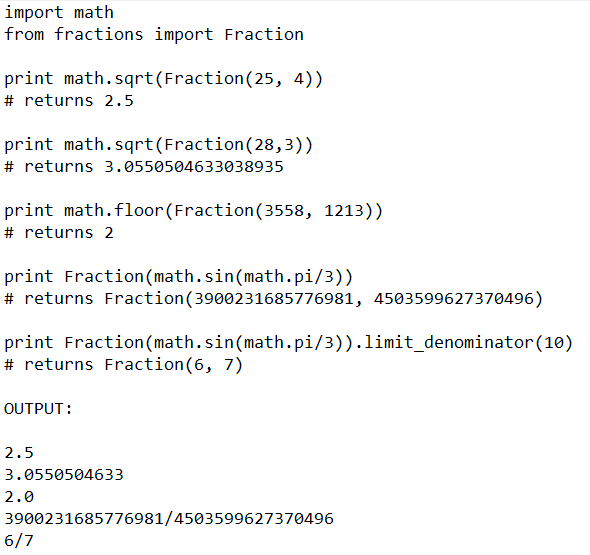Calculating Fractions With Python

1. The basics

The fraction class is found in Lib/fractions.py so to import:

from fractions import Fraction

There are a number of ways to instantiate the Fraction class. First, you can pass in the numerator and the denominator.

>>> Fraction(1, 2)

Fraction(1, 2)

Or instantiate with another fraction:

>>> f = Fraction(1, 2)

>>> Fraction(f)

Fraction(1, 2)

Instantiate with a float:

>>> Fraction(2.5)

Fraction(5, 2)

Or with a decimal:

>>> from decimal import Decimal

>>> Fraction(Decimal('1.1'))

Fraction(11, 10)

Lastly, and probably the most interesting - you can instantiate with a string:

>>> Fraction('9/16')

Fraction(9, 16)

Ultimately, the Fraction class is designed so that there is very little processing you have to do before instantiating the class. It knows how to deal with multiple data types.

2. Automatically reduces

Reducing isn't that hard, but for some complicated fractions, it can take a few steps. The Fraction class is especially helpful because it automatically reduces your fraction.

>>> Fraction(153, 272)

Fraction(9, 16)

You might not be able to reduce 153/172 in your head, but the Fraction class will do it quickly.

3. Binary operations

You can perform binary operations on a Fraction just like you can ints or floats!

>>> Fraction(1, 2) + Fraction(3, 4)

Fraction(5, 4)

So that's pretty great, but you can also mix in integers or floats. But as you might expect - adding an integer returns a Fraction object while adding a float returns a float.

>>> Fraction(5, 16) + 3

Fraction(53, 16)

>>> Fraction(5, 16) + 3.0

3.3125

Here are some examples of other binary operations:

>>> Fraction(5, 16) - Fraction(1, 4)

Fraction(1, 16)

>>> Fraction(1, 16) * Fraction(3, 16)

Fraction(3, 256)

>>> Fraction(3, 16) / Fraction(1, 8)

Fraction(3, 2)

Now let's try with exponentiation:

>>> Fraction(1, 8) ** Fraction(1, 2)

0.3535533905932738

It returns a float probably because the fraction is impossible to calculate within reason. We can actually use the limit_denominator method to get a semi-accurate Fraction as a result.

>>> f = Fraction(1, 8) ** Fraction(1, 2)

>>> Fraction(f).limit_denominator()

Fraction(235416, 665857)

Keep in mind you can mix in strings and other data types upon instantiation:

>>> Fraction("1/2") + Fraction(2.0)

Fraction(5, 2)

>>> Fraction(2) * Fraction(" 1/2 ")

Fraction(1, 1)

4. Accessing the attributes of a Fraction

So you've got a Fraction and you've done some calculations, now how do we access the attributes?

Without reading the documentation you might try Fraction.numerator and Fraction.denominator and you'd be right.

>>> f = Fraction(221, 234) + Fraction(1, 2)

>>> f.numerator

13

>>> f.denominator

9

Or print the entire fraction as a string:

>>> print f

13/9

>>> a = str(f)

>>> a

'13/9'